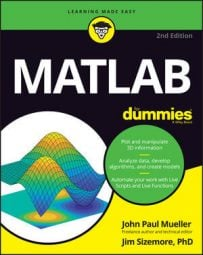##### MATLAB For DummiesMultiplication occurs at several different levels in MATLAB. Here, the process is broken down to the act of matrix multiplication so that you can see each level as it progresses.

## Multiplying two vectors

Vectors are just matrices of only one row or column. Remember that you create a row vector by separating values using a comma, such as [1, 2]. To create column vectors, you use a semicolon, such as [3; 4]. You can also use prime to create a row or column vector. For example, [3, 4]’ is equivalent to [3; 4].

When you want to multiply one vector by another, you must have one row and one column vector. Try it for yourself by typing d = [1, 2] * [3; 4] and pressing Enter. You get the value 11 for output.

Of course, the method used to perform the multiplication is to multiply the first element in the row vector by the first element of the column vector, and add the result to the multiplication of the second element of the row vector and the second element of the column vector. What you end up with is d = 1 * 3 + 2 * 4. This form of multiplication is also called an inner product.

It’s also possible to create an outer product using MATLAB. In this case, each element in the first vector is multiplied by every element of the second vector, and the results of each multiplication are placed in a separate element.

To put this in perspective, you’d end up with a 2 x 2 matrix consisting of [1 * 3, 2 * 3; 1 * 4, 2 * 4]. The easiest way to see how this works is by trying it yourself. Type e = bsxfun(@times, [1, 2], [3; 4]) and press Enter. You see

```e =
3  6
4  8```

The bsxfun() function performs element-by-element operations. You supply a function nam to perform an element-by-element math operation on two objects. You can use the @times function name, which performs multiplication. The two inputs are a row vector and a column vector.

The output is a 2 x 2 matrix where the row 1 column 1 element is 1 * 3. Likewise, the row 1 column 2 element is 2 * 3. The second row multiplication works the same way as the first.

Another way to obtain the outer product is to ensure that the column vector appears first. For example, type e = [3; 4] * [1, 2] and you receive an output of

```e =
3  6
4  8```

## Multiplying a matrix by a vector

When performing multiplication of a matrix by a vector, the order in which the vector appears is important. Row vectors appear before the matrix, but column vectors appear after the matrix. To see how the row vector approach works, type f = [1, 2] * [3, 4; 5, 6] and press Enter. You see an output of

```f =
13 16```

The first element is produced by 1 * 3 + 2 * 5. The second element is produced by 1 * 4 + 2 * 6. However, the number of elements in the matrix must agree with the number of elements in the vector.

To see how this works, type g = [1, 2, 3] * [4, 5; 6, 7; 8, 9] and press Enter. The result is

```g =
40 46```

The number of elements in the output is controlled by the matrix in this case. For example, if the matrix were to have three elements in each row, the output would also have three elements. To see this principle in action, type h = [1, 2, 3] * [4, 5, 6; 7, 8, 9; 10, 11, 12] and press Enter. The result is

```h =
48 54 60```

Working with a column vector is similar to working with a row vector, except that the position of the vector and matrix are exchanged. For example, if you type i = [4, 5, 6; 7, 8, 9; 10, 11, 12] * [1; 2; 3] and press Enter, you see this result:

```i =
32
50
68```

Notice that the output is a column vector instead of a row vector. The result is produced by these three equations:

```1 * 4 + 2 * 5 + 3 * 6
1 * 7 + 2 * 8 + 3 * 9
1 * 10 + 2 * 11 + 3 * 12```

The order of the multiplication differs because you’re using a column vector instead of a row vector.

## Multiplying two matrices

When working with matrices, the number of rows in the first matrix must agree with the number of columns in the second matrix. To see this for yourself, type j = [1, 2, 3; 4, 5, 6] * [7, 8; 9, 10; 11, 12] and press Enter. You see the output as

```j =
58 64
139 154```

The output of the first column, first row is defined by 1 * 7 + 2 * 9, + 3 * 11. Likewise, the output of the second column, first row is defined by 1 * 8 + 2 * 10 + 3 * 12. The matrix math works just as you would expect.

Order is important when multiplying two matrices. You can create the same two matrices, but obtain different results depending on order. If you reverse the order of the two matrices in the previous example by typing k = [7, 8; 9, 10; 11, 12] * [1, 2, 3; 4, 5, 6] and pressing Enter, you obtain an entirely different result:

```k =
39 54 69
49 68 87
59 82 105```

In this case, the output of the first column, first row is defined by 7 * 1 + 8 * 4. Likewise, the output of the second column of the first row is defined by 7 * 2 + 8 * 5.